## Friday, April 06, 2007

### The Shock Model: A Review (Part I)

This story was initially published on TheOilDrum.

WebHubbleTelescope, a long time TOD poster, has been one of the most active in the blogosphere in the area of oil production modeling. He has advocated a more physically based approach instead of a heuristic curve fitting approach such as the Hubbert Linearization. He proposed an original method, the so called Shock Model, that has a clear physical interpretation and that is making use of both the production profile and the discovery data. I think that a review of the Shock Model is long overdue.

I also propose three modifications or extensions:

1. Originally, the instantaneous extraction rate function E(t) has to be provided by the user. I propose a method to estimate E(t) directly from the observed production profile.
2. Reserve growth is modeled as a fourth convolution function based on an empirical parabolic cumulative growth functions (this will be detailed in part II).
3. A new way to project future extraction rate (in part II).

In summary, the shock model is a simple and intuitive model that is making use of both the production profile and the discovery curve. In this essay, the method is applied on the world conventional crude oil production (crude oil + condensate) and the ASPO backdated discovery data. Interestingly, the derived Reserve to Production ratio (R/P) seems to match the values obtained when using the proven reserve numbers (BP) once corrected for Middle-East spurious reserve revisions (in 1985, 1988 and 1990). In addition, R/P values are presently at a record low levels and below what have been observed during the previous oil shocks.

The code in R language is provided at the end of this post.

### From Discovery to Reserves

The Shock Model is described in details on Mobjectivist. It is based on the following three equations: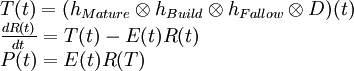where:

 D Discovery (backdated) T Instantaneous gross reserve addition R Oil reserves E Extraction rate or shock function P Annual oil production hBuild,hFallow,hMature Exponential functions

The first equation will create reserve additions from the initial discovery numbers (backdated). The second equation is the reserve evolution equation and dictates how reserves will change over time from the cumulative addition of new reserves and consumption. The three filtering functions involved in the computation of the instantaneous reserve addition T(t) are exponential distribution functions: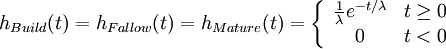where λ is the mean time period implied by each process. The main reason behind this filtering process is to simulate the different steps (Fallow, Build and Maturation) involved in developing an oil field as shown on Figure 1.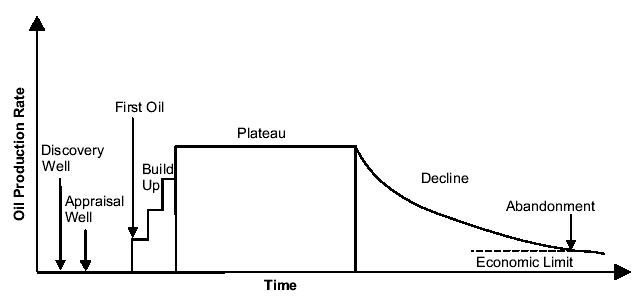Fig 1. Timeframe for the development of an oil field1.

The result of the three convolution operations is equivalent to one convolution with a Gamma distribution: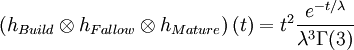On Figure 2, we can see an original discovery impulse is being spread out on a large time period.Fig 2. The filtering operation by three successive Exponential
distributions is equivalent to a Gamma distribution

The convolution by a Gamma function will smooth and shift the original discovery curve by 2λ. Using the ASPO backdated discovery curve (Figure 3), we get a shifted and smooth reserve curve.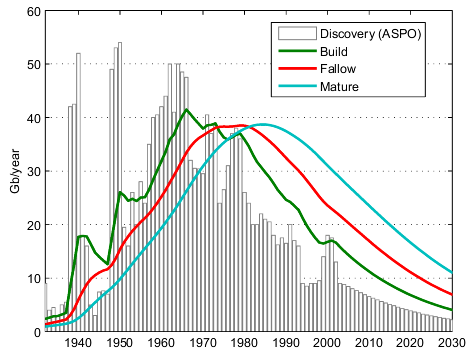Fig 3. Effect of the succesive convolutions on the original backdated
discovery curves (ASPO) .

The question is how to choose a correct value for λ. WebHubbleTelescope is proposing λ=8 years. Higher values for λ will increase the degree of smoothing and the time lag between peak discovery and peak reserves. If you compare the simulated reserve curve with the actual proven reserve numbers (Figure 4), you can see that for λ=3 the reserve curve is matching closely the proven reserve numbers (once corrected for Middle-East increase).Fig 4. Simulated oil reserves R for different values of
λ.

### Estimating the shock function

In reality, the extraction rate E is not a constant and presents large fluctuations especially during oil shocks. In WHT's original method, the shock function E(t) is set manually which is not an easy task in my opinion. I propose to estimate the shock function by literally tracking the instantaneous oil production using a bootstrap filter. I have previously applied this technique with good results. In a nutshell, the boostrap filter find the optimal value for E(t) at each time t by stochastically exploring a set of possible values and weighting them according to how well observed production levels are predicted (the technical term is Sequential Importance Resampling or SIR). A result of this approach for λ=3 years is shown on Figure 5, we can see how closely the production levels are reproduced by the model.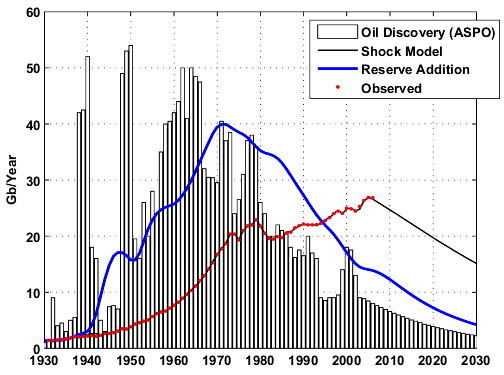Fig 5.
World production for crude oil + condensate2.

The figure below is the estimated shock function E(t) with the proposed technique. The shock function is assumed constant in the future and equals to the last estimated value.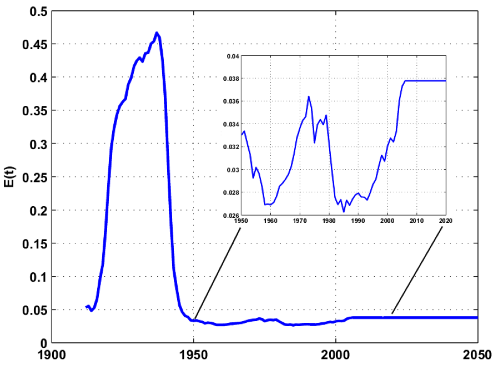Fig 6. Shock function E(t) estimated by the bootstrap filter (with λ= 3 years).
Click to enlarge.

### Forecasting ability

In my opinion, the model has limited predictive abilities because it cannot predict correctly future extraction rate values (i.e. the shock function E is unknown in the future). WHT has made the following prediction for crude oil + NGL.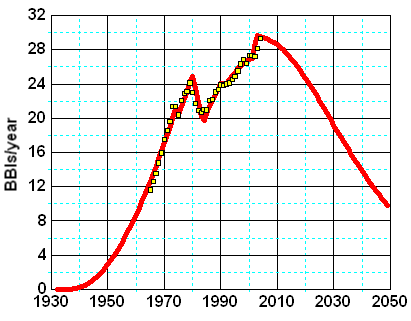Fig 7. World production forecast (crude oil + NGL) .

This forecast includes NGL production which I don't think is appropriate because the model is based on conventional oil discoveries. On Figure 8, we can see the application of the shock model for different time intervals assuming that the extraction rate remains constant in the future and equals to the last estimated value. Predicted production levels are falling immediately because E(t) should increase in order to maintain or increase production. In part II, I will propose a simple solution to deal with this problem.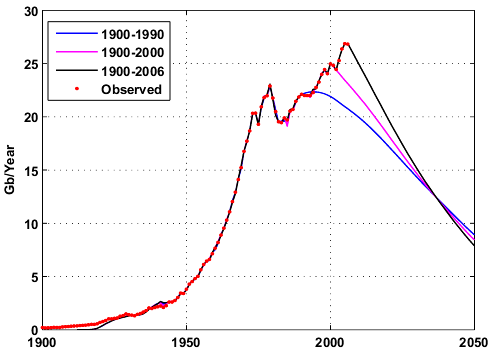Fig 8. Different world production forecasts for crude oil + condensate .

It's interesting to compare the inverse of the estimated extraction rate (1/E(t)), which is equal to the reserve to production ratio (R/P), and fluctuations in nominal crude oil prices:

1. When R/P values are below 30 years, prices are increasing. This was the case in 1970 at the time when demand for oil was very strong and energy efficiency not part of the vocabulary. A lowering of consumption after 1985 (mainly a combination of economic slowdown, energy conservation, drilling frenzy,...) has contributed in an increase in the R/P values.
2. The recent increase in price since 2003 corresponds also to current R/P values below the 30 years threshold minimum (27.6 years) observed in the 70s.
3. The ASPO discovery data is probably underestimating some significant amount of reserve growth that has occured in the late 90s hence the premature fall of the simulated R/P curve around 2000.
4. The R/P values given by the model are surprisingly closed to the R/P computed from proven reserves (BP) once corrected from large reserve additions in middle-east countries (red circles).
5. In order for the R/P value to come back above 30 years, either reserves need to increase significantly or crude oil consumption needs to decrease significantly.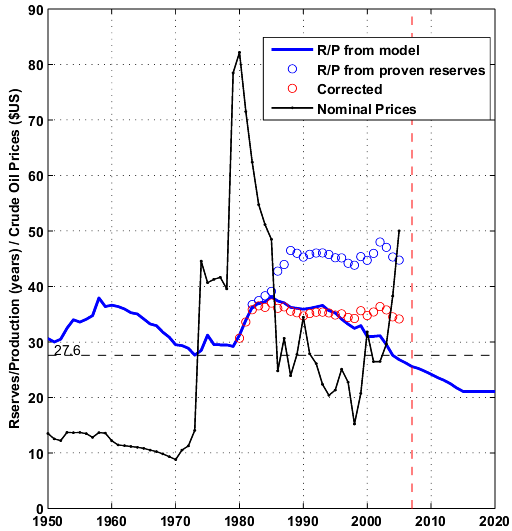Fig 9. Crude oil prices are inflation adjusted (nominal prices). The red dotted vertical line is the year
2007.

In Part II, I will look at the modelisation of reserve growth and a new way to improve the predictive ability of the shock model.

#### Footnotes:

1Giant oil fields and their importance for future oil production, Fredrik Robelius, PhD Thesis.
2The data for the world production of crude oil + condensate is composed of:
• 1900-1959: API Facts and Figures Centennial edition 1959.
• 1960-2006: EIA data.

#### Code:

The code is available in R language, the windows version of R software is available here. You will need to install the Matlab package (go in "Packages/Install Package(s)" then choose a CRAN site and select Matlab from the package list). To execute the program, open the file given below and click on "Edit/Run all".

Code